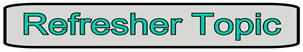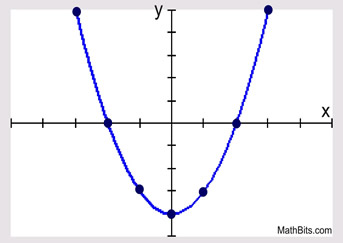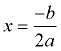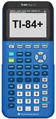Graphing Quadratic Functions MathBitsNotebook.com Terms of Use   Contact Person: Donna RobertsWhen you first learned to graph straight lines, you did so by preparing a table (or chart) of x-values and y-values to obtain points on the graph, and you plotted these points. Since you were dealing with straight lines, with constant (never changing) slopes, only a few points (actually only two) were needed to produce the line.

A similar approach is used for graphing quadratic functions. These graphs, however, can seldom be drawn properly by plotting only two or three points because the slope of a parabola is not constant. More points are needed to uncover the shape of the graph (a parabola) and its location on the set of coordinate axes.Let's take a look at chart graphing for a quadratic function.
Graph y = x2 - 4:
A chart is prepared using both positive and negative values for x. Since we were not given any information as to what values of x to use in the chart, we are "guessing" at which values will tell us the entire story about this graph.
 x x2 - 4 y -3 (-3)2 - 4 5 -2 (-2)2 - 4 0 -1 (-1)2 - 4 -3 0 (0)2 - 4 -4 1 (1)2 - 4 -3 2 (2)2 - 4 0 3 (3)2 - 4 5The points are plotted to reveal the graph. Be sure to draw a "smooth" curved graph. Do not play "connect the dots" by drawing straight line segments between the points. There are no straight line segments in a parabola, as its slope is never constant.

We were lucky in choosing the set {-3, -2, -1, 0, 1, 2, 3} for the x-values in our chart.
These x-values reveal pertinent information about the graph:
opening upward, vertex (turning point) at (0,-4), and roots (zeros) at (-2,0) and (2,0).

Remember: The roots (zeros) are the poiChoosing Chart Values: To guarantee that the x-values you choose for your chart will show pertinent information about the graph, start by finding the axis of symmetry of the parabola from its equation. The axis of symmetry is a vertical line through the vertex, with equation. Use this x-value as the "middle" x-value in the chart and choose 3 (or more) values less than, and greater than, this value.

nts where the graph crosses the x-axis.Quadratic functions can also be graphed from vertex form.
See Vertex Form of Quadratic Function to see how to quickly graph from vertex form.Quadratic functions can also be graphed from vertex form using rate of change.
See Quadratic Function - Rate of Change to see another method of graphing.Using the graphing calculator to graph quadratic functions.

You can find pertinent information regarding a parabola using a graphing utility, such as a graphing calculator. If you need help with your graphing calculator, use the link at the right.For calculator help with graphing parabolas click here."Solving" Quadratic Equations by Graphing:
Using a graph to determine the roots (x-intercepts) of a quadratic equation may be a difficult process. If you are graphing by hand, it may be hard to find the exact x-intercepts (the roots), especially when the x-intercepts are not integer values. If you must rely on graphing to find the roots of a quadratic equation, use a graphing utility with the capability of finding (or giving a decimal approximation for) the x-intercepts or (roots).For calculator help with quadratic equations click here.